# Mechanical Engineering - Hydraulics and Fluid Mechanics

### Exercise :: Hydraulics and Fluid Mechanics - Section 6

11.

The most efficient section of a channel is

 A. triangular B. rectangular C. square D. trapezoidal

Answer: Option D

Explanation:

No answer description available for this question. Let us discuss.

12.

According to Bernoulli's equation

 A.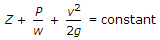B.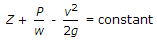C.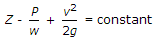D.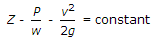Answer: Option A

Explanation:

No answer description available for this question. Let us discuss.

13.

The ratio of the inertia force to the elastic force is called

 A. Reynold's number B. Froude's number C. Weber's number D. Mach number

Answer: Option D

Explanation:

No answer description available for this question. Let us discuss.

14.

An opening in the side of a tank or vessel such that the liquid surface with the tank is below the top edge of the opening, is called

 A. weir B. notch C. orifice D. none of these

Answer: Option B

Explanation:

No answer description available for this question. Let us discuss.

15.

The total pressure on the top of a closed cylindrical vessel of radius (r) completely filled up with liquid of specific weight (w) and rotating at (ω) rad/s about its vertical axis, is

 A.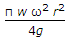B.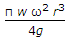C.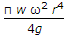D.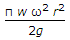Answer: Option C

Explanation:

No answer description available for this question. Let us discuss.

#### Current Affairs 2021

Interview Questions and Answers